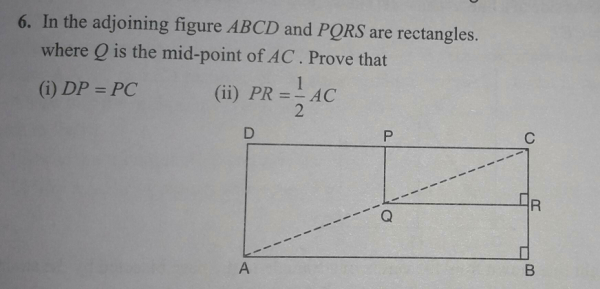"
">

# In the figure below..."

Solution:

Given ABCD and PQRS are rectangles.

AC is diagonal and Q is the midpoint of AC.

So AQ = QC

(i)

AD || PQ In triangle ACD.

By Basic proportionality theorem

DP/PC = AQ/QC = 1/1

So DP = PC

(ii)

We know AQ = QC = 1/2 AC

In the rectangle PQRS, diagonals PR, and QC are equal in length and bisect each other.

So PR = QC = 1/2 AC or

PR = 1/2 AC

Updated on: 10-Oct-2022

23 Views